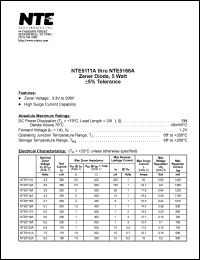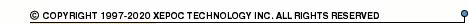More than12 563 440 queries processedNTE515 series datasheets. Manufacturer: NTE.

 NTE5111A Zener diode, 5 watt, +-5% tolerance. Nominal zener voltage Vz = 3.3V. Test current Izt = 380mA. in 2-pin -- package. Operational temperature range from -65°C to 200°C. Datasheet*) NTE5112A Zener diode, 5 watt, +-5% tolerance. Nominal zener voltage Vz = 3.6V. Test current Izt = 350mA. in 2-pin -- package. Operational temperature range from -65°C to 200°C. Datasheet*) NTE5113A Zener diode, 5 watt, +-5% tolerance. Nominal zener voltage Vz = 3.9V. Test current Izt = 320mA. in 2-pin -- package. Operational temperature range from -65°C to 200°C. Datasheet*) NTE5114A Zener diode, 5 watt, +-5% tolerance. Nominal zener voltage Vz = 4.3V. Test current Izt = 290mA. in 2-pin -- package. Operational temperature range from -65°C to 200°C. Datasheet*) NTE5115A Zener diode, 5 watt, +-5% tolerance. Nominal zener voltage Vz = 4.7V. Test current Izt = 260mA. in 2-pin -- package. Operational temperature range from -65°C to 200°C. Datasheet*) NTE5116A Zener diode, 5 watt, +-5% tolerance. Nominal zener voltage Vz = 5.1V. Test current Izt = 240mA. in 2-pin -- package. Operational temperature range from -65°C to 200°C. Datasheet*) NTE5117A Zener diode, 5 watt, +-5% tolerance. Nominal zener voltage Vz = 5.6V. Test current Izt = 220mA. in 2-pin -- package. Operational temperature range from -65°C to 200°C. Datasheet*) NTE5118A Zener diode, 5 watt, +-5% tolerance. Nominal zener voltage Vz = 6.0V. Test current Izt = 200mA. in 2-pin -- package. Operational temperature range from -65°C to 200°C. Datasheet*) NTE5119A Zener diode, 5 watt, +-5% tolerance. Nominal zener voltage Vz = 6.2V. Test current Izt = 200mA. in 2-pin -- package. Operational temperature range from -65°C to 200°C. Datasheet*) NTE5120A Zener diode, 5 watt, +-5% tolerance. Nominal zener voltage Vz = 6.8V. Test current Izt = 175mA. in 2-pin -- package. Operational temperature range from -65°C to 200°C. Datasheet*) NTE5121A Zener diode, 5 watt, +-5% tolerance. Nominal zener voltage Vz = 7.5V. Test current Izt = 175mA. in 2-pin -- package. Operational temperature range from -65°C to 200°C. Datasheet*) NTE5122A Zener diode, 5 watt, +-5% tolerance. Nominal zener voltage Vz = 8.2V. Test current Izt = 150mA. in 2-pin -- package. Operational temperature range from -65°C to 200°C. Datasheet*) NTE5123A Zener diode, 5 watt, +-5% tolerance. Nominal zener voltage Vz = 8.7V. Test current Izt = 150mA. in 2-pin -- package. Operational temperature range from -65°C to 200°C. Datasheet*) NTE5124A Zener diode, 5 watt, +-5% tolerance. Nominal zener voltage Vz = 9.1V. Test current Izt = 150mA. in 2-pin -- package. Operational temperature range from -65°C to 200°C. Datasheet*) NTE5125A Zener diode, 5 watt, +-5% tolerance. Nominal zener voltage Vz = 10V. Test current Izt = 125mA. in 2-pin -- package. Operational temperature range from -65°C to 200°C. Datasheet*) NTE5126A Zener diode, 5 watt, +-5% tolerance. Nominal zener voltage Vz = 11V. Test current Izt = 125mA. in 2-pin -- package. Operational temperature range from -65°C to 200°C. Datasheet*) NTE5127A Zener diode, 5 watt, +-5% tolerance. Nominal zener voltage Vz = 12V. Test current Izt = 100mA. in 2-pin -- package. Operational temperature range from -65°C to 200°C. Datasheet*) NTE5128A Zener diode, 5 watt, +-5% tolerance. Nominal zener voltage Vz = 13V. Test current Izt = 100mA. in 2-pin -- package. Operational temperature range from -65°C to 200°C. Datasheet*) NTE5129A Zener diode, 5 watt, +-5% tolerance. Nominal zener voltage Vz = 14V. Test current Izt = 100mA. in 2-pin -- package. Operational temperature range from -65°C to 200°C. Datasheet*) NTE5130A Zener diode, 5 watt, +-5% tolerance. Nominal zener voltage Vz = 15V. Test current Izt = 75mA. in 2-pin -- package. Operational temperature range from -65°C to 200°C. Datasheet*) NTE5131A Zener diode, 5 watt, +-5% tolerance. Nominal zener voltage Vz = 16V. Test current Izt = 75mA. in 2-pin -- package. Operational temperature range from -65°C to 200°C. Datasheet*) NTE5132A Zener diode, 5 watt, +-5% tolerance. Nominal zener voltage Vz = 17V. Test current Izt = 70mA. in 2-pin -- package. Operational temperature range from -65°C to 200°C. Datasheet*) NTE5133A Zener diode, 5 watt, +-5% tolerance. Nominal zener voltage Vz = 18V. Test current Izt = 65mA. in 2-pin -- package. Operational temperature range from -65°C to 200°C. Datasheet*) NTE5134A Zener diode, 5 watt, +-5% tolerance. Nominal zener voltage Vz = 19V. Test current Izt = 65mA. in 2-pin -- package. Operational temperature range from -65°C to 200°C. Datasheet*) NTE5135A Zener diode, 5 watt, +-5% tolerance. Nominal zener voltage Vz = 20V. Test current Izt = 65mA. in 2-pin -- package. Operational temperature range from -65°C to 200°C. Datasheet*) NTE5136A Zener diode, 5 watt, +-5% tolerance. Nominal zener voltage Vz = 22V. Test current Izt = 50mA. in 2-pin -- package. Operational temperature range from -65°C to 200°C. Datasheet*) NTE5137A Zener diode, 5 watt, +-5% tolerance. Nominal zener voltage Vz = 24V. Test current Izt = 50mA. in 2-pin -- package. Operational temperature range from -65°C to 200°C. Datasheet*) NTE5138A Zener diode, 5 watt, +-5% tolerance. Nominal zener voltage Vz = 25V. Test current Izt = 50mA. in 2-pin -- package. Operational temperature range from -65°C to 200°C. Datasheet*) NTE5139A Zener diode, 5 watt, +-5% tolerance. Nominal zener voltage Vz = 27V. Test current Izt = 50mA. in 2-pin -- package. Operational temperature range from -65°C to 200°C. Datasheet*) NTE5140A Zener diode, 5 watt, +-5% tolerance. Nominal zener voltage Vz = 28V. Test current Izt = 50mA. in 2-pin -- package. Operational temperature range from -65°C to 200°C. Datasheet*) NTE5141A Zener diode, 5 watt, +-5% tolerance. Nominal zener voltage Vz = 30V. Test current Izt = 40mA. in 2-pin -- package. Operational temperature range from -65°C to 200°C. Datasheet*) NTE5142A Zener diode, 5 watt, +-5% tolerance. Nominal zener voltage Vz = 33V. Test current Izt = 40mA. in 2-pin -- package. Operational temperature range from -65°C to 200°C. Datasheet*) NTE5143A Zener diode, 5 watt, +-5% tolerance. Nominal zener voltage Vz = 36V. Test current Izt = 30mA. in 2-pin -- package. Operational temperature range from -65°C to 200°C. Datasheet*) NTE5144A Zener diode, 5 watt, +-5% tolerance. Nominal zener voltage Vz = 39V. Test current Izt = 30mA. in 2-pin -- package. Operational temperature range from -65°C to 200°C. Datasheet*) NTE5145A Zener diode, 5 watt, +-5% tolerance. Nominal zener voltage Vz = 43V. Test current Izt = 30mA. in 2-pin -- package. Operational temperature range from -65°C to 200°C. Datasheet*) NTE5146A Zener diode, 5 watt, +-5% tolerance. Nominal zener voltage Vz = 47V. Test current Izt = 25mA. in 2-pin -- package. Operational temperature range from -65°C to 200°C. Datasheet*) NTE5147A Zener diode, 5 watt, +-5% tolerance. Nominal zener voltage Vz = 51V. Test current Izt = 25mA. in 2-pin -- package. Operational temperature range from -65°C to 200°C. Datasheet*) NTE5148A Zener diode, 5 watt, +-5% tolerance. Nominal zener voltage Vz = 56V. Test current Izt = 20mA. in 2-pin -- package. Operational temperature range from -65°C to 200°C. Datasheet*) NTE5149A Zener diode, 5 watt, +-5% tolerance. Nominal zener voltage Vz = 60V. Test current Izt = 20mA. in 2-pin -- package. Operational temperature range from -65°C to 200°C. Datasheet*) NTE5150A Zener diode, 5 watt, +-5% tolerance. Nominal zener voltage Vz = 62V. Test current Izt = 20mA. in 2-pin -- package. Operational temperature range from -65°C to 200°C. Datasheet*) NTE5151A Zener diode, 5 watt, +-5% tolerance. Nominal zener voltage Vz = 68V. Test current Izt = 20mA. in 2-pin -- package. Operational temperature range from -65°C to 200°C. Datasheet*) NTE5152A Zener diode, 5 watt, +-5% tolerance. Nominal zener voltage Vz = 75V. Test current Izt = 20mA. in 2-pin -- package. Operational temperature range from -65°C to 200°C. Datasheet*) NTE5153A Zener diode, 5 watt, +-5% tolerance. Nominal zener voltage Vz = 82V. Test current Izt = 15mA. in 2-pin -- package. Operational temperature range from -65°C to 200°C. Datasheet*) NTE5154A Zener diode, 5 watt, +-5% tolerance. Nominal zener voltage Vz = 87V. Test current Izt = 15mA. in 2-pin -- package. Operational temperature range from -65°C to 200°C. Datasheet*) NTE5155A Zener diode, 5 watt, +-5% tolerance. Nominal zener voltage Vz = 91V. Test current Izt = 15mA. in 2-pin -- package. Operational temperature range from -65°C to 200°C. Datasheet*) NTE5156A Zener diode, 5 watt, +-5% tolerance. Nominal zener voltage Vz = 100V. Test current Izt = 12mA. in 2-pin -- package. Operational temperature range from -65°C to 200°C. Datasheet*) NTE5157A Zener diode, 5 watt, +-5% tolerance. Nominal zener voltage Vz = 110V. Test current Izt = 12mA. in 2-pin -- package. Operational temperature range from -65°C to 200°C. Datasheet*) NTE5158A Zener diode, 5 watt, +-5% tolerance. Nominal zener voltage Vz = 120V. Test current Izt = 10mA. in 2-pin -- package. Operational temperature range from -65°C to 200°C. Datasheet*) NTE5159A Zener diode, 5 watt, +-5% tolerance. Nominal zener voltage Vz = 130V. Test current Izt = 10mA. in 2-pin -- package. Operational temperature range from -65°C to 200°C. Datasheet*) NTE5160A Zener diode, 5 watt, +-5% tolerance. Nominal zener voltage Vz = 140V. Test current Izt = 8mA. in 2-pin -- package. Operational temperature range from -65°C to 200°C. Datasheet*) NTE5161A Zener diode, 5 watt, +-5% tolerance. Nominal zener voltage Vz = 150V. Test current Izt = 8mA. in 2-pin -- package. Operational temperature range from -65°C to 200°C. Datasheet*) NTE5162A Zener diode, 5 watt, +-5% tolerance. Nominal zener voltage Vz = 160V. Test current Izt = 8mA. in 2-pin -- package. Operational temperature range from -65°C to 200°C. Datasheet*) NTE5163A Zener diode, 5 watt, +-5% tolerance. Nominal zener voltage Vz = 170V. Test current Izt = 8mA. in 2-pin -- package. Operational temperature range from -65°C to 200°C. Datasheet*) NTE5164A Zener diode, 5 watt, +-5% tolerance. Nominal zener voltage Vz = 180V. Test current Izt = 5mA. in 2-pin -- package. Operational temperature range from -65°C to 200°C. Datasheet*) NTE5165A Zener diode, 5 watt, +-5% tolerance. Nominal zener voltage Vz = 190V. Test current Izt = 5mA. in 2-pin -- package. Operational temperature range from -65°C to 200°C. Datasheet*) NTE5166A Zener diode, 5 watt, +-5% tolerance. Nominal zener voltage Vz = 200V. Test current Izt = 5mA. in 2-pin -- package. Operational temperature range from -65°C to 200°C. Datasheet*) NTE515 2.5A Iout, 800V Vrrm Fast Recovery Rectifier Datasheet*) NTE551 1.0A Iout, 1.5kV Vrrm Fast Recovery Rectifier Datasheet*)